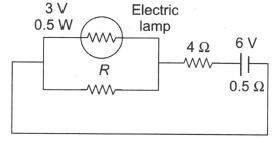What should be the value of resistance R in the circuit shown in figure so that the electric lamp consumes the rated power?

# What should be the value of resistance R in the circuit shown in figure so that the electric lamp consumes the rated power?1. A

$4.5\text{\hspace{0.17em}}\Omega$

2. B

$6\text{\hspace{0.17em}}\Omega$

3. C

$12\text{\hspace{0.17em}}\Omega$

4. D

None of these

Register to Get Free Mock Test and Study Material

+91

Live ClassesRecorded ClassesTest SeriesSelf Learning

Verify OTP Code (required)

### Solution:

The electric lamp consumes the rated power if the voltage across electric lamp is 3 V.

$\therefore \text{\hspace{0.17em}\hspace{0.17em}\hspace{0.17em}}\mathrm{resistance}\text{\hspace{0.17em}\hspace{0.17em}}\mathrm{across}\text{\hspace{0.17em}}\mathrm{AB}\text{\hspace{0.17em}\hspace{0.17em}}\mathrm{is}\text{\hspace{0.17em}}4.5\text{\hspace{0.17em}\hspace{0.17em}}\mathrm{\Omega }$

Resistance of lamp, ${\mathrm{R}}_{\mathrm{b}}=\text{\hspace{0.17em}\hspace{0.17em}\hspace{0.17em}}\frac{{\mathrm{V}}^{2}}{{\mathrm{P}}_{\mathrm{b}}}\text{\hspace{0.17em}}=\text{\hspace{0.17em}}\frac{{3}^{2}}{0.5}=\text{\hspace{0.17em}}18\text{\hspace{0.17em}}\mathrm{\Omega }$

$\frac{{\mathrm{RR}}_{\mathrm{b}}}{{\mathrm{R}}_{\mathrm{b}}}=4.5\text{\hspace{0.17em}}\mathrm{\Omega }\text{\hspace{0.17em}};\text{\hspace{0.17em}\hspace{0.17em}}18\text{\hspace{0.17em}}\mathrm{R}\text{\hspace{0.17em}}=\text{\hspace{0.17em}}\frac{9}{2}\text{\hspace{0.17em}\hspace{0.17em}}\left(18+\mathrm{R}\right)\text{\hspace{0.17em}}⇒\text{\hspace{0.17em}\hspace{0.17em}}\mathrm{R}=6\text{\hspace{0.17em}}\mathrm{\Omega }$